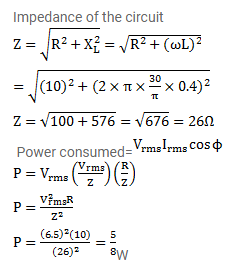# A coil has a resistance of 10Ω and an inductance of 0.4 henry.

Question:

A coil has a resistance of $10 \Omega$ and an inductance of $0.4$ henry. It is connected to an Ac source of $6.5 \mathrm{~V}$, $\frac{30}{\pi} \mathrm{Hz}$. Find the average power consumed in the circuit.

Solution: